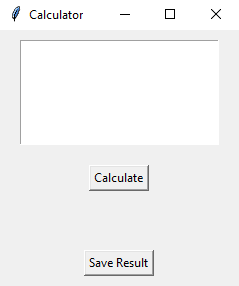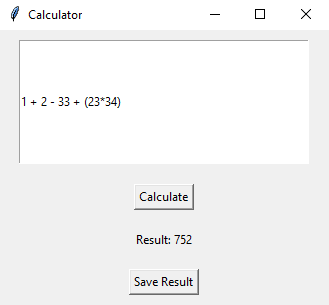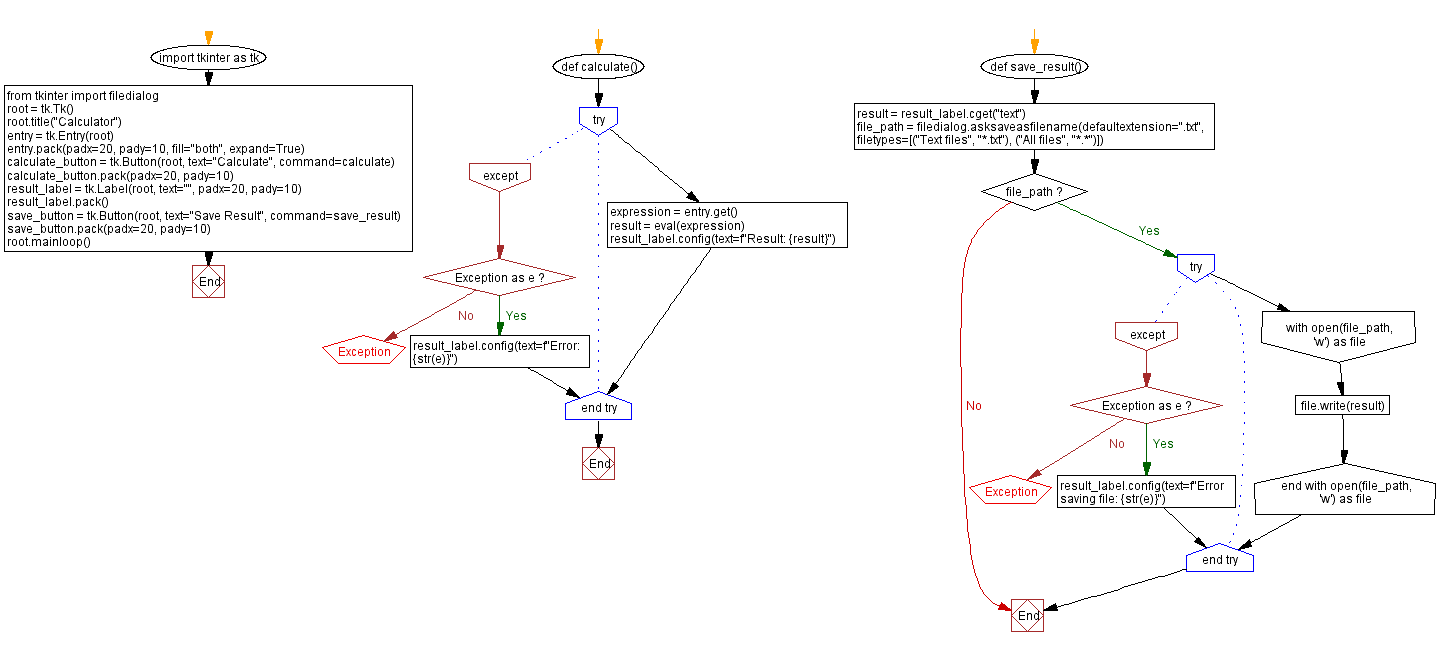﻿ Python Tkinter calculator with file saving

# Python Tkinter calculator with file saving

## Python Tkinter Dialogs and File Handling: Exercise-13 with Solution

Write a Python program to implement a simple calculator application that performs calculations and saves the results to a text file using file dialogs. Use Tkinter.

Sample Solution:

Python Code:

``````import tkinter as tk
from tkinter import filedialog

def calculate():
try:
expression = entry.get()
result = eval(expression)
result_label.config(text=f"Result: {result}")
except Exception as e:
result_label.config(text=f"Error: {str(e)}")

def save_result():
result = result_label.cget("text")
file_path = filedialog.asksaveasfilename(defaultextension=".txt", filetypes=[("Text files", "*.txt"), ("All files", "*.*")])
if file_path:
try:
with open(file_path, 'w') as file:
file.write(result)
except Exception as e:
result_label.config(text=f"Error saving file: {str(e)}")

root = tk.Tk()
root.title("Calculator")

entry = tk.Entry(root)

calculate_button = tk.Button(root, text="Calculate", command=calculate)

result_label.pack()

save_button = tk.Button(root, text="Save Result", command=save_result)
root.mainloop()
```
```

Explanation:

In the exercise above -

• Import "tkinter" as "tk" and "filedialog" for creating GUI components and opening file dialogs.
• Define the calculate() function, which attempts to evaluate the expression entered in the Entry widget. If the evaluation succeeds, it appears on the Result Label. If the expression is incorrect, it displays an error message.
• Define the save_result() function, which saves the results displayed in the Result Label to a text file. It uses "filedialog.asksaveasfilename()" to open a file dialog for specifying the file path and writes the result to the selected file.
• Create the main Tkinter window, set its title, and create an Entry widget for entering calculations.
• Create a "Calculate" button that calls the calculate() function when clicked.
• Create a Result Label to display the calculation result.
• Create a "Save Result" button that calls the "save_result()" function when clicked.
• The main event loop, root.mainloop(), starts the Tkinter application.

Sample Output:

``````
``````

Flowchart:Python Code Editor:

What is the difficulty level of this exercise?

Test your Programming skills with w3resource's quiz.

﻿

## Python: Tips of the Day

Summing a sequence of numbers (calculating the sum of zero to ten with skips):

```>>> l = range(0,10,2)
>>> sum(l)
20
```

We are closing our Disqus commenting system for some maintenanace issues. You may write to us at reach[at]yahoo[dot]com or visit us at Facebook